DIRECTION : Jetha lal works ‘a’ hours a day and rests ‘b’ hours a day. This pattern continues for 1 week, with an exactly opposite pattern next week, and so on for four weeks. Every fifth week he has a different pattern. When he works longer than he rests, his wage per hour is twice what he earns per hour when he rests longer than he works. The following are his daily working hours for the weeks numbered 1 to 13. It is also known that Jetha lal receives Rs.20 per hour of work when he works longer than he rests in the day.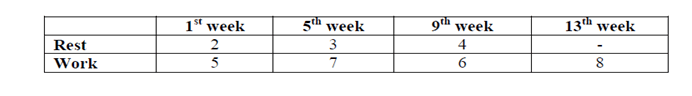A week consists of six days and a month consists of 4 weeks.

Question 1. What is his salary for the 1st month?
(a) Rs.1760
(b) Rs.1440
(c) Rs.1320
(d) Rs.1680

Question 2. Jetha lal’s average monthly salary at the end of the first four months will be
(a) Rs.1780
(b) Rs.2040
(c) Rs.1830
(d) Rs.1680

Question 3. The new manager Babita stipulated that Rs.5 is deducted for every hour of rest and Rs. 25 is paid per hour starting from 9th week, then what will be the change in Jetha lal’s salary for the 3rd month? (Hourly deductions are constant forall weeks starting 9th week)
(a) Rs.540
(b) Rs.480
(c) Rs.240
(d) Rs.120

Question 4. Using the data in the previous questions, what will be the total earning of Jethalal at the end of sixteen weeks?
(a) Rs.7320
(b) Rs.7800
(c) Rs.8400
(d) Rs.9600

From the first week data we can arrive at the following work pattern of Jetha lal for the 1st month.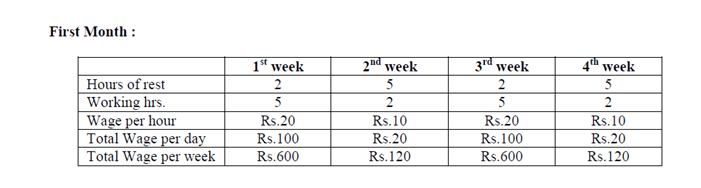Thus his total wage = (600+120+600+120) = Rs.1440

Let us compile the data for second, third and fourth month.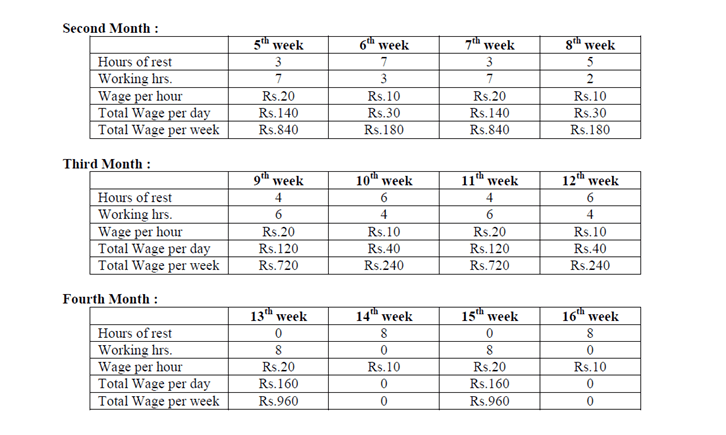Total wage for 1st month = Rs.1440

Total wage for 2nd month = (840+180+840+180) = Rs.2040

Total wage for 3rd month = (720+240+720+240) = Rs.1920

Total wage for 4th month = (960+960) = Rs.1920

Total wage for the 4 months = (1440+2040+1920+1920) = 7320

Hence the average salary = 7320/4 = Rs.1830

Using the above data, we can revise the wage compilation for the third month as given below: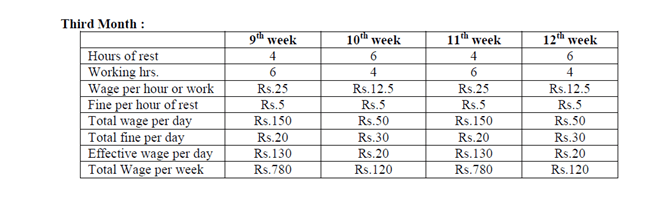So now his third month age = (780+120+780+120) = Rs.1800.

Previously he used to earn Rs.1920 in the third month.

Hence change in Jetha lal’s salary for the 3rd month = (1920 – 1800) = Rs.120.

For the fourth month, the new wage compilation will be as given below: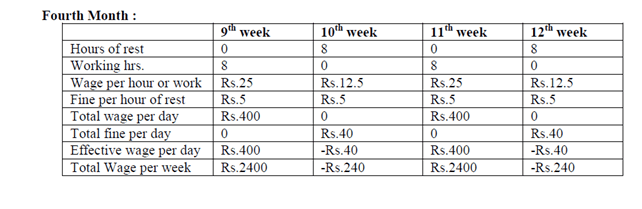So now his total wage for the 4th month = (2400+2400-240-240) = Rs.4320.

Since the calculations for the first two months are made as per the old scheme of things, this has already been computed.

Total wage for 1st month = Rs.1440

Total wage for 2nd month = Rs.2040

Calculation for the third and fourth month are as per new calculations and they are :

Total wage for 3rd month = Rs.1800

Total wage for 4th month = Rs.4320

So total salary for the four months = (1440+2040+1800+4320) = Rs.9600.